# Free Quant Trading Interview Guide

This guide is the property of

CanaryWharfian.co.uk, and any replication or reselling of this guide without the consent of the owners of the aforementioned site is strictly prohibited.

Question 4. You are given a six sided die. What is the expected value of the di￿erence between the two dice rolls?

Solution

Denote by X1 the ￿rst throw and by X2 the second one. Denote D = X1 ￿ X2. Then

E(D) = E(X1 ￿ X2) = E(X1) ￿ E(X2) = 0:

Question 5. You roll two dice and then multiply the outcome. What is the probability of getting a product which is a perfect square?

Solution

We only need to ￿nd the probability that the second throw is equal to the ￿rst one. No matter what the ￿rst throw gives, this is always 1=6.

Question 6. You roll three dice. What is the probability of getting a sum of ten?

Solution

We could solve this as a cobinatorics problem (see e.g. prob. 7 below), but here's another way: denote the three throws as X1; X2 and X3 and the sum of n throws as Sn. Then

1

P (S3 = 10) = 6 fP (S2 = 9) + P (S2 = 8) + : : : + P (S2 = 4)g :

This means that to reach 10 at the third throw means that we have either gotten 9 at the second throw and then have probability 1=6 to throw 1 or that we got an eight at second throw and have probability 1=6 to get two etc. Then we have

 P (S2 = 9) = 1 fP (S1 = 6) + : : : + P (S1 = 3)g = 4 6 62

and similarly P (S2 = 8) = 5=62, P (S2 = 7) = 1=6, P (S2 = 6) = 5=62, P (S2 = 5) = 4=62, P (S2 = 4) = 3=62 and we get

 P (S3 = 10) = 1 (4 + 5 + 6 + 5 + 4 + 3) = 1 : 3 8 6

Question 7. Given a twelve sided die, you roll the die repeatedly until the cumulative sum is odd. What is the number of the cumulative sum you can have with the highest probability?

Solution

Suppose we throw N times. We must ￿nd the probability that the N ￿1 throws are even and the last one is odd. Denote the cumulative sum by

 : N￿1 N X X SN = 2 Xn + 2XN ￿ 1 = 2 Xn ￿ 1; n=1 n=1

2

where the Xn corresponds to the n:th throw but with Xn 2 [1; : : : ; 6] (since 2Xn is even).

 Then (2 n=1 Xn ￿ 1 = q) (n=1 Xn = z) P (SN = q) = 12￿N # = 12￿N # ; N N X X

where #fg denotes the number of cases inside the brackets and z = probability that we get the odd number q is

 p(q) =: 1 P (SN = q) = 1 12￿N # ( N Xn = z) X X X

q+12 2 Z+. Then the

:

 N=1 N=1 n=1 N Now we need to count the number of ways to get n=1 Xn = z for Xn 2 [1; : : : ; 6] and z z21 X ￿ combinatorics problem with the solution ￿ Z+. For n 1 this would be a fairly simple P ￿ n ￿ N￿1 ￿ n ! ￿ N￿1 n ! n . ￿ ￿ For X 7 we can just map to the previous problem by X X + 6, which corresponds to z z 6N, which results in z￿6N￿1 . To get the solution for 1 X 6, we just subtract the latter from the ￿rst, which results in ￿ ￿ 1 1 ￿ N 1 ￿ N=1 ￿N 1￿ ￿ N=1 (1) p(q) = 12￿N z ￿ 1 12￿N z ￿ 6N ￿ 1 X ￿ X ￿ = 1 13 z (z 1)! 1 12￿N z ￿ 6N ￿ 1 : (2) 13 ￿12 ￿ ￿ ￿ N=1 ￿ N ￿ 1 ￿ X

Note that the ￿rst term grows fast and the second term only kicks in at z > 6 (although the binomial coe￿cient is formally de￿ned for negative values, here we assume it to be zero for negatives). We can already guess here that the maximum probably occurs at z = 6, i.e. q = 11, since at z = 7 the second term is ￿1=12. Indeed, p(11) ￿ 0:124 and p(13) ￿ 0:135 ￿ 0:083 ￿ 0:052 and the probability decays rapidly for larger values. Therefore 11 is the most likely outcome.

Question 8. Given a thirty-sided die, you roll the die repeatedly until the cumulative sum is greater than or equal to 300. What is the most likely result of the sum?

Solution

Suppose that at the last throw I got 300. Then the previous throw could have been

270; 271; : : : ; 299, which is total 30 possibilities. Suppose I got 301. Then the previous throw

could have been 271; : : : ; 299, a total of 29 possibilities and so on. So 300 is the most likely outcome.

Question 9. You have a thirty-sided and I have a twenty-sided die. We roll both of them and whoever gets the higher roll wins. If we roll the same amount I win. The loser pays the dollar amount of the winning roll.

3

A)What is the probability that I win?

B)What is the expected value of this game for you?

C)How does this value change if I have the option to re-roll once?

D)What is a fair price for me to get such an option?

E)If such an option would cost zero dollars, how many of them would I need to in order to be in favor of the game?

Solution

A)

Denote by X a roll of the thirty-sided die and by Y the twenty-sided die. Then

 1 30 20 1 30 7 X X X P (Y ￿ X) = 20 ￿ 1fm ￿ ng = 20 ￿ 30 m = 20 ￿ 0:35; 30 n=1 n=1 m=1

where 1 is the indicator function.

B)

We can de￿ne the payout function for us as

:

F (X; Y ) = X 1fX > Y g ￿ Y 1fX ￿ Y g

and just take the expectation:

 1 30 20 E (F (X; Y )) = X X 20 ￿ 30 [n 1fn > mg ￿ m 1fn ￿ mg] n=1 m=1 1 20 30 1 20 m = X X n ￿ X X 20 ￿ 20 ￿ 30 30 m m=1 n=m+1 m=1 n=1 = 51 ￿ 10:2 5

(3)

(4)

(5)

C)

Now we can roll up to two times, so de￿ne the Z = maxfY1; Y2g as the opponent's result, where the Yi are the throws of the 20 sided die. The probability distribution for Z can be de￿ned as

4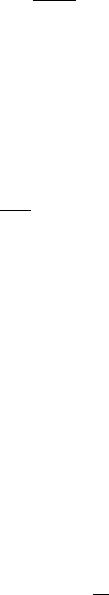:

P (Z = z) = E (1fmax(Y1; Y2) = zg)

 1 20 X 1fmax(n; m) = zg = 202 n;m=1 1 20 n 1 20 20 X X X X = ￿n;z + ￿m;z 400 n=1 m=1 400 n=1 m=n+1

= 2z400￿ 1:

Then the payo￿ function is

:

F (X; Z) = X 1fX > Zg ￿ Z 1fX ￿ Zg

and the expectation is computed similarly as in B:

 (F (X; Z)) = 1 30 20 2m ￿ 1 [n 1 n > m m 1 n m ] E X X f g ￿ f ￿ g 30 n=1 m=1 400

= 1381300 ￿ 4:6

(6)

(7)

(8)

(9)

(10)

(11)

D)

The fair price is now just the di￿erence with the expected result without a re-roll and with re-roll, i.e. 10:2 ￿ 4:6 = 5:6.

E)

For N rerolls, my expected winnings are 10:2 ￿4:6N. The smallest integer for which this is negative is N = 3, so three such options would be needed for the opponent to be in favor of the game.

Question 10. I give you a twelve-sided die and will pay you whatever the die lands on. If you are unhappy with the roll, you can choose to roll another two six-sided dice and I will pay you the sum of the two dice. How much are you willing to pay to play this game?

Solution

Denote A as the single throw of the twelve-sided die and by B the throw of the two six side dice. The expectations of the two types of throws are

12

E (A) = 121 Xn = 6:5

n=1

and

E (B) = 2 ￿ 3:5 = 7:

5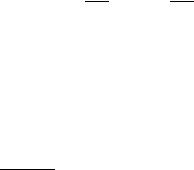Since the latter expectation is 7, my strategy is such that every time I roll a six or less with the twelve-sided die, I choose to roll the two dice. Otherwise I will stick with what I've got. Then the expected result of the game is

E(Game) = P (A ￿ 6) E (B) + P (A > 6) E (AjA > 6)

 = 1 ￿ 7 + 1 ￿ 7 + 8 + 9 + 10 + 11 + 12 = 8:25; 2 2 6

which is the fair price of the game.

Question 11. You are given a hundred-sided die. Every time you roll you can choose to either get paid the dollar amount of that roll or pay one dollar for one more roll. What is the expected value of the game?

Solution

Denote the roll of the die as X. The expected value of a single throw of the die is

100

E(X) = 1001 Xn = 1012 = 50:5

n=1

so the expected value of a throw with a reroll is 49.5. The strategy is therefore such that if I get 49 or lower, I will choose to pay the dollar and roll again. Otherwise I will stick to what I've got. Then the expected value of the game is

E(game) = P (X ￿ 49) (E(X) ￿ 1) + P (X ￿ 50) E (XjX ￿ 50)

 = 49 ￿ 49:5 + 51 1 100 n = 62:5: 100 nX 100 100 =50

Question 12. You and I play a game with two dice, one ten-sided and one six-sided. You guess the sum of the numbers after we roll them. If your guess is correct, you get the sum of the numbers in dollars, else you get nothing. How would you make the best guess?

Solution

Denote a roll of the ten sided and six sided dice respectively as X and Y . The probability P (X +Y = n) is just the number of possible combinations of X +Y = n divided by the total number of combinations, which is 60. From 1 to 7 the number of combinations is the same as with two six-sided dice, but to get 8, the possible pairs are (X; Y ) = (7; 1); (6; 2); : : : ; (2; 6), i.e. total 6 combinations. Similarly, to get 9 we have pairs (8; 1); (7; 2); : : : ; (3; 6), which gives 6 combinations etc. up to total sum of 11. To get 12, we have pairs (10; 2); (9; 3); : : : ; (6; 6), which is total 5 pairings, and the number just decreases for totals higher than this. So the total number of combinations is (0; 1; 2; 3; 4; 5; 6; 6; 6; 6; 6; 5; 4; 3; 2; 1) for a total sum of (1; 2; : : :). The highest probabilities occur for 7,8,9,10 and 11, so we should choose 11 to maximize our winnings.

6:
pn =
￿6￿
n

Question 13. Is it possible to change the numbers on two six-sided dice to other positive numbers so that the probability distribution of their sum remains unchanged?

Solution

Question 14. If you toss three coins, what is the probability of getting at least two heads?

Solution

Number of all possible head, tail combinations is 23 = 8. The number of ways of choosing two heads and one tail is ￿32￿ = 3 and then we have the case of three heads, which gives the ￿nal probability

P= 3 + 1 = 1:

8 2

Question 15. If you toss a coin six times, what is the expected number of heads?

Solution 1 (brute force)

This is a nice exercise in combinatorics, although not as clever as the second method below...

The total number of possibilities is 26. The number of ways of choosing n heads is , which gives the probability distribution for the number of heads,

￿ ￿

n6 2￿6

and the expected number of heads can be computed by reorganizing the sum over the bino- mial coe￿cients as follows:

 6 6 6 E = n=0 npn = n=0 n￿n￿2￿6 = 2￿66! n=0 (6 n)!n! X X 6 X n ￿ = 2￿66! 6 1 = 2￿66! 5 1 = 6 5 5! 2￿5 X ￿ ￿ Xk ￿ X ￿ (6 n)!(n 1)! (5 k)!k! 2 (5 k)!k! n=1 =0 k=0

5￿ ￿

=3 Xn n5 2￿5 = 3

n=0

Solution 2 (symmetry)

Suppose the expected number of heads is EH = X. Because it's equally likely to get a tail than a head, we have ET = EH. On the other hand, the expected number of heads plus tails must be six, i.e. EH + ET = 2EH = 6, so EH = 3.

7Question 16. If you drop four coins on the table, given that one is heads, what is the probability that the rest are tails?

Solution

Question 17. You ￿ip four coins and I give you a dollar for each heads. How much would you pay to play this game?

B)What if I give you the option to re-￿ip one of the coins?

C)What if I have the option to re-￿ip one of the coins? Solution

Denote by X the number of heads. Denote pn = P (X = n) = ￿n4￿2￿4. Expected payo￿ for this game is then

4 ￿ ￿

E(game) = X n4 2￿4 = 2

n=0

B)

Now my strategy is such that if there's at least one tails, toss that again and if I have all heads, do nothing. The expected payo￿ of this strategy is then

:

E = P (X = 4) ￿ 4 + P (X = 3)E(XjX ￿ 3)+

: : : + P (X = 2)E(XjX ￿ 2) + P (X = 1)E(XjX ￿ 1) + P (X = 0)E(XjX ￿ 0):

Now E(XjX ￿ n) = n + 1=2, and the expression is just

E = 164 + 161 12 + 164 32 + 166 52 + 164 72 ￿ 2:47:

C)

By symmetry, my expected payo￿ is now instead reduced by the amount it increased in part B), i.e.

E￿ 2 ￿ 0:47 = 1:53:

Question 18. You ￿ip a coin as many times as needed until either a pattern of HHT or HTT occurs. What is the probability that HHT occurs before HTT?

Solution

Suppose we ￿rst get H. If on the second ￿ip we get a T, then on a third ￿ip the game ends with another T or is essentially reset with another H, i.e. a sequence HTH, since TH is

8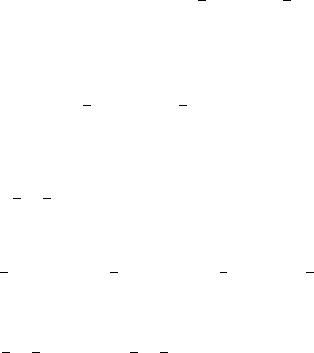not the start of an either one of the sequences. Suppose now that the second ￿ip is H, then on a third ￿ip the game ends with a T, but does not reset if we get a H, i.e. HHH, since there's no way to get to HTH from this sequence before a HHT occurs! So we can conlude that HHT is more likely to occur ￿rst.

To ￿nd the probability, denote by A the event that HHT occurs before HTT. Then by the total probability formula, we can decompose

P (A) = P (AjH)P (H) + P (AjT )P (T ) = 12P (AjH) + 12P (AjT );

where P (AjH) denotes the sequence HHT given that the ￿rst ￿ip was H. Now starting with a T is same as starting all over, so P (AjT ) = P (A) and therefore P (A) = P (AjH). We can again decompose

P (AjH) = 12P (AjHT ) + 12P (AjHH):

Note that P (AjHH) = 1, because there's no way to get to HTT ￿rst starting with a HH. Then

12 + 12P (AjHT ) = P (AjH) = P (A):

Again decompose:

P (AjHT ) = 12P (AjHT H) + 12P (AjHT T ) = 12P (AjH) = 12P (A);

since HTT is obviously already the other sequence and HTH is equivalent to starting with a

H. Then we have

12 + 12P (AjHT ) = 12 + 14P (A) ￿ P (A); which has a solution P (A) = 2=3.

Question 19. How many ways can you get three heads on ￿ve coins? B) Two heads in a row?

Solution

Question 20. You ￿ip a coin. You stop ￿ipping after three heads in a row occur. What is the expected number of ￿ips?

Solution

Denote the single ￿ip by X and by En the expected number of ￿ips until we get n heads in a row. We can calculate E1 as follows: we have a probability 1=2 that we get a head and 1=2 that we don't. If we don't get a head, we need to ￿ip again, which adds 1 to the expectation. Formally,

9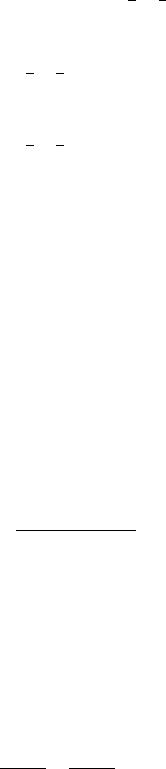1 1

E1 = P (X = H) + P (X = T )(E1 + 1) = 2 + 2(E1 + 1); which gives E1 = 2. To get E2, we have ￿rst ￿ipped on average E1 times, so

1 1

E2 = E1 + 2 + 2(E2 + 1);

which gives E2 = 6 and ￿nally, to get E3 we have

1 1

E3 = E2 + 2 + 2(E3 + 1);

which gives E3 = 14.

Question 21. You ￿ip a coin thirteen times. What is the expected product of number of heads and number of tails?

Solution

Denote the number of heads and tails respectively by Nh and Nt. Flipping 13 times, we have Nt = 13 ￿ Nh. The probability that we get n heads is P (Nh = n) = ￿13n ￿2￿13. Then by

a direct computation,

13￿ ￿

E(NhNt) = 2￿13 X(13n ￿ n2) 13n :

n=0

Here is a general formula for calculating moments of the binomial distribution, which will come handy in later problems:

 N (n n!K)! ￿n ￿ 2￿N n=0 X N ￿

N

= 2￿N N! X 1

(N ￿ n)!(n ￿ K)!

n=K

 N￿K ￿ 1 ￿ = 2￿N N! X n=0 (N K n)!n! ￿ ￿ = 2￿K N! 2￿(N￿K) N￿K N ￿ K = 2￿K N! ; n=0 n (N ￿ K)! (N ￿ K)! X ￿ (n￿2)! N ￿ ￿ and P because 2￿N n=0 Nn = 1 for all N. We can now write 13n ￿ n2 = 12n ￿ n(n ￿ 1) = 12n n! use the above formula to get E (N N ) = 12 ￿ 13 ￿ 12 ￿ 13 = 39: h t 2 4

Question 22. I toss N+1 coins. You toss N coins. You win if you have at least as many heads as I do. What is the probability that you win?

10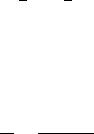Solution

Consider one of the other guy's coin. If it is a head, then he wins if he gets at least as many heads in the remaining coins as you do. If it is a tail, then you win if you have at least as many heads as he does. This is a symmetrical situation, so the probability that you win is 1=2

Question 23. You start out with X amount of money and we play a game. We toss a coin and you lose twenty percent of your money if a head appears, and gain the same amount if a tail appears. Why do you never get exactly X amount of money in your pocket after playing this game repeatedly?

Solution

To get back to X after losing 20% n times and winning 20% m times, we need to solve the equation

￿4￿n ￿6￿m

5 5

= 1

for positive integers n; m. Take the natural logarithm on each side, and the equation becomes

n log(4=5) + m log(6=5) = 0;

which simpli￿es to

n= log(6=5) =: q: m log(5=4)

What this says is that there is a solution if q is a rational number, which it is not (q = 0:817059:::), so there is no solution.

Question 24. You ￿ip a coin four times. If you at ￿rst ￿ip a head, you win one dollar. If you ￿ip a consecutive head, you win double your previous winnings. What is the expected value of your winnings?

Solution

The probability that I will get n consecutive heads is just pn := 2￿n. The payo￿ after n succesfull ￿ips of consecutive heads is fn := 2n￿1. Then the expected winnings are

 4 4 X X E := fnpn = 2n￿12￿n = 2: n=1 n=1

Question 25. You throw ninety-nine coins and I throw a hundred. What is the probability that you will have more heads than I do?

11

Solution

Denote by A the r.v. of the number of heads for the 99 throws and by B the 100 throws. Then P (A > B) = P (A ￿ B) ￿ P (A = B). We know from problem 22 that P (A ￿ B) = 1=2. On the other hand the probability that you get n heads with 99 throws is the same as the probability that you get 99 ￿ n heads because the coin is fair. Then

 99 99 X X P (A = B) = P (A = n \ B = n) = P (A = 99 ￿ n \ B = n) n=0 ￿ 99 n=0 = P (A + B = 99) = ￿2￿199 ￿ 0:056: 199

Therefore P (A > B) ￿ 0:444:

Question 26. You ￿ip four coins. You get one dollar each time a head appears, what is the expected payo￿?

B)What if you know for sure that at least two heads will appear?

C)What if you can choose to either keep all of the ￿ips or re-￿ip all four coins? Solution

Denote by X the number of heads, so that pn = P (X = n) = ￿n4￿2￿4, which is just the number of ways of getting n heads and 4￿n tails divided by the total number of possibilities, 24. Then the expected payo￿ is

4 ￿ ￿

E1 := E(Payo￿) = Xn n4 2￿4 = 2

n=0

B)

We know there will always be two heads, and the expected winnings for a remaining two-dice game is 1, so

E2 := E(Payo￿) = 3:

C)

Because the regular game's payo￿ is 2, Our strategy here is such that if we get total 1 head or less, we re-￿ip all the coins and the re-￿ip has a payo￿ equal to E1. Then the payo￿ is

E3 := P (X ￿ 1)E1 + P (X ￿ 2)E(Payo￿jX ￿ 2):

As it happens, E(Payo￿jX ￿ 2) = E2, so we have

 E3 = 1 + 4 ￿ 2 + + 6 + 4 + 1 ￿ 30 = 5 = 2:5: 16 16 11 2

12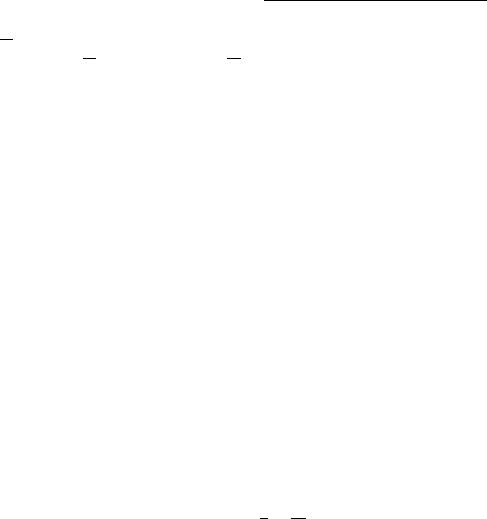Question 27. You have ￿ve coins, and one of them is double-headed. You pick one coin at random without looking and ￿ip it ￿ve times. Suppose that the outcome is ￿ve heads. What is the probability that the coin picked is the double-headed one?

Solution

Denote by D the event of picking the double headed coin and by 5H the event of getting 5 heads with 5 ￿ips. Then by the Bayes theorem,

 P (D 5H) = P (5H D) P (D) = P (5HjD)P (D) ; P (5H) j j P (5HjD)P (D) + P (5HjD)P (D)

where D is the complement of D, i.e. the probability of not picking D. We have P (5HjD) = 1, P (D) = 1=5, P (D) = 4=5 and P (5HjD) = 2￿5. Therefore

 P (Dj5H) = 1=5 = 8 ￿ 0:89: 1=5 + 2￿54=5 9

Question 28. You and I play a game where we start at zero and ￿ip a coin until someone wins. Every heads that are ￿ipped add plus one to the number and every tails add minus one. If the number reaches minus ten I win, although if it reaches twenty then you win. What is the probability I will win?

Solution

Suppose we are at position x. Denote the probability that the game will end up at ￿10 before 20 when starting at x by px. We know that one ￿ip before being at x, we must have been at either x ￿ 1 or at x + 1 with equal probability, so

 1 1 px = px￿1 + px+1: 2 2

We also know that p￿10 = 1 and p20 = 0. The problem is therefore to solve the above linear di￿erence equation with the aforementioned boundary conditions (you can compare this to solving a linear di￿erential equation). It's easy to check that there is a linear solution of the form px = a + bx. Solving for the boundary conditions gives the equations a ￿ 10b = 1 and a + 20b = 0 which give the solutions a = 2=3 and b = ￿1=30 and therefore

2x

px = 3 ￿ 30;

so p0 = 2=3, which is the desired answer.

Question 29. You and I play with two decks of cards: one has thirteen reds and thirteen blacks in it, the other has twenty-six reds and twenty-six blacks. You select one of the two decks, and then draw two cards from it. If both cards are black, you win. Which pack will you choose?

13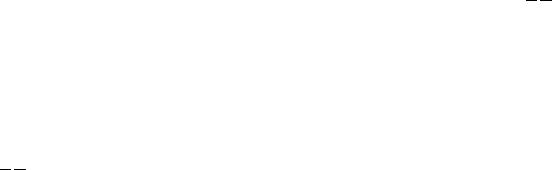Solution

Suppose I draw from the 26 card pack. The probability of getting two blacks with two draws is 1326 1225 = 12 1225 . Drawing from the 52 card pack gives the probability 2652 2551 = 12 2551 . Since 25=51 > 12=25, we conclude that it's best to choose the 52 card pack.

Question 30. You are sitting in front of a roulette table with a six-sided die and a standard deck of playing cards. What is the probability that you play all three games and receive the same number in all three?

Solution

We can get numbers 0 : : : 36 in the roulette, numbers 1 : : : 6 in the die game and numbers

1 : : : 13 when drawing the cards, all with uniform distributions. The order in which this game is played, so we can assume we start withe die. Suppose then we get any number between 1 : : : 6. The probability that all the numbers are same is then just the probability that both the roulette and cards give the number obtained from the die game, i.e. p = 131 371 ￿ 0:002.

Question 31. You have a deck of cards in which aces are excluded. What is the probability of taking the ￿rst two cards and both of them being six?

Solution

In the ￿rst draw I can draw any of the four sixes out of total 48 cards, and on the second draw there are only three sixes out of the remaining 47 cards, so the probability is

4 3 ￿ 0:005.

48 47

Question 32. You have a deck of cards. What is the expected number of cards you have to draw to get cards of all four suites?

Solution

Question 33. If you have a deck of cards and start putting them in another stack, how many cards do you have to put down to guarantee a Three of a Kind?

Solution

Before drawing the card that will guarantee a Three of a Kind, the deck must include all the numbers from 1 to 13 exactly twice, which is total 26 cards. Therefore we will necessarily have a Three of a Kind in a deck of 27 cards.

Question 34. You are playing Russian Roulette. In the chamber there are four blanks and two bullets. If someone shoots a blank next to you, would you spin or take another shot?

14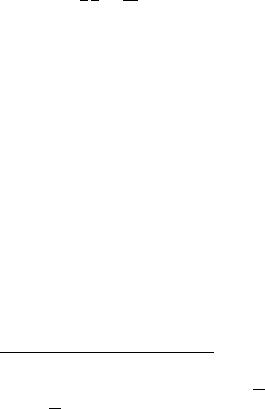Solution

After one blank shot, there are two bullets left and three blank positions, if the revolver is not spun again. Therefore the probability that the next shot results in a "brainstorm" is 2=5. Spinning again, the probability is just 1=3, so it's de￿nitely a good idea to spin again.

Question 35. You have a square, and place three dots along the four edges at random. What is the probability that the dots, when connected, do not form a triangle? B) What is the probability that the dots lie on distinct edges?

Solution

The only way a triange cannot be formed is if all the dots lie on the same edge. The probability of this occurring is

P (all 3 on the same edge) = 1 ￿ 14 14 = 161 ;

since the ￿rst dot can be on any edge. B)

The ￿rst dot can be on any edge, so the second one can be only on the remaining three edges and the last one at the remaining two edges. The probability is thus 1 ￿ 44 24 = 3=8.

Question 36. You have three pancakes. One is with both sides burned, one is with one side burned, the last one with no sides burned. You combined them in a plate, and the top side is burned. What is the probability of the other side is burned?

Solution

We can denote the pancakes as A = (1; 1), B = (1; 0) and C = (0; 0) where 1 denotes a burned side and 0 a non-burned side. Also denote by 'tsb' that the top side is burned. We are asking for the probability of choosing A given that the other side is observed to be burned. By the Bayes theorem,

 P (A tsb) = P (tsb A) P (A) = P (tsbjA)P (A) : P (tsb) j j P (tsbjA)P (A) + P (tsbjA)P (A)

We have P (tsbjA) = 1, since both sides of A are burned, P (A) = 1=3 = 1 ￿ P (A), since there are three pancakes to choose from, and P (tsbjA) = 1=4, since that is the probability we pick B and that it is placed burned side up. Therefore,

 P (Ajtsb) = 1=3 = 2 : 31 + 41 32 3

Question 37. If there is a thirty percent chance of rain on Monday and a thirty percent chance of rain on Tuesday, what is the probability that it rains at least once during the ￿rst two days of the week?

15Solution

The probability that it will rain at least once is equal to one minus the probability that it will not rain on either day, so the total probability is

1 ￿ (107 )2 = 10051 :

Question 38. A survey is given to all passengers on a number of di￿erent planes. The survey asks each person how full their plane was. If ￿fty percent of people claim that their plane was eighty percent full, while the other ￿fty percent claim that their plane was twenty percent full, how full was the average plane?

Solution

Note that the solution is not (80% + 20%)2 = 50%, since the planes that are 80% and 20% full have di￿erent numbers of passengers in them. Suppose then that there are two kinds of planes with 100 seats. If a person says that his plane was 80% full, then that plane had 80 passengers in it. If a person says that her plane was 20% full, then that plane had 20 passengers in it. To get a 50/50 response rate of 80% and 20%, we need to compare an equal number of people, i.e. one plane of 80 passengers and four planes of 20 passengers and compute the average out of the ￿ve planes, which is

(80 + 4 ￿ 20)=5 = 32;

so the average plane was 32% full.

Question 39. You know you have an eighty percent chance of seeing at least one shooting star over the next hour. What is your chance of seeing a shooting star over the next half hour?

Solution

Denote by p the probability of observing a shooting star over a half an hour period.

 The probability of not observing a shooting star over the one hour period is then (1 ￿ p)2 , 2 which must be equal to 0:2, so we must solve the equation (1 ￿ p) = 1=5, which gives p

p = 1 ￿ 1= 5 ￿ 0:55.

Question 40. If the probability of seeing at least one car in an hour is 544/625, what's the probability of seeing no cars in a ￿fteen minute interval?

Solution

Denote by p the probability of observing a car over a ￿fteen minute interval. The prob- ability of not observing a car over a ￿fteen minute interval is then (1 ￿ p)4, which must be equal to 1 ￿ 544=652 = 81=652, so we must solve the equation (1 ￿ p)4 = 81=652 = (3=5)4, which gives p = 1 ￿ 3=5 = 2=5.

16

Question 41. There are ￿fty noodles in a bowl. You can tie two ends of either one noodle or two di￿erent noodles, forming a nod. After ￿fty nods are tied, what is the expected value of number of circles in the bowl?

Question 42. You dip a cube into paint and pull it out. Then you cut it into twenty-seven sub-cubes and put them in a bag. If you randomly pick a sub-cube out the bag and roll it on the table, what is the probability that no paint is visible?

Question 43. I have one red and ten white balls. I have to put all the balls into two jars. Then I pick one jar in random and pick a ball random. How should I put the balls into two jars so that I have the highest chance of picking the red ball?

Question 44. How much would you pay for a one in a thousand chance to win one million dollars?

Question 45. A dealer is selling you a car whose value is uniformly distributed between zero and a thousand, but you do not know the real value and you need to bid for the car. If your bid price is higher than the value of the car, the deal will be done at your bid price and you can afterwards resell the car elsewhere for 1.5 times its real value. Otherwise, the deal will not be done. You can only bid once. What will be your optimal bid price?

Question 46. There is a box that contains two hundred coins and X of them are heads. You get paid X if you own the box. The only other person in the market has the advantage of getting to see the ￿rst 20 coins before you both end up bidding on the box. How much do you bid?

Question 47. You have a lottery ticket with ten slots. Behind each slot there is an equally distributed number between zero and one and your payout is the maximum number between any of these slots. How much are you prepared to pay for the lottery ticket?

Question 48. You play a game where you roll a hundred-sided die. You can either get paid the amount rolled in dollars or pay a dollar to roll again. You can always choose to pay another dollar to roll again, until you are ￿nally satis￿ed with your current roll and get paid out based on that. What is the optimal strategy for this game and what is your expected winnings given this strategy?

Question 49. I give you a hundred blank cards, and you can write a single positive integer on each card. I look at the cards when you are done, then I shu￿e the deck. I guess the top card of the deck, and if I am right, I make the dollar which is written on the card. What numbers should you write on the cards to minimize the expected return of mine?

Question 50. You have two functions. The ￿rst returns an integer between zero and ten, while the second returns any real value between zero and ten. If you get paid based on whatever value is returned, which payo￿ function would you rather have?

17

Question 51. You and I play a game where a random number between one and twenty is chosen. One of you will pick a number and then the other will pick a di￿erent number. The one that guesses the closer number wins X dollars. Should you choose to go ￿rst, and what number should you choose?

Question 52. I have a hundred slips of paper in a box, with numbers one through a hundred on them. You pay a dollar for the opportunity to pick a slip of paper out of the box. At this point, you can choose to end the game and get paid the amount listed on the slip of paper. If you choose not to end the game, then you put the slip of paper you just drew back into the box, pay another dollar to pick out another slip of paper, and decide once again if you would like to get paid or keep going. Make me a ￿ve-point market on the value of this game.

Question 53. Suppose you ￿ip a fair coin ten times. Consider the product of the number of heads and the number of tails. Make me as thin of a market as you can on the expected value of this quantity.

Question 54. How many ways are there of writing twenty as the sum of odd integers, where the order does not matter? Make a market on the size of the answer.

Question 55. Make me a two-point wide market on the chance that a randomly selected number between one and a hundred does not contain a seven.

Question 56. What is the expected number of ￿ips of a coin to simulate a six-sided die?

Question 57. Simulate a scenario where I can get a fair coin from the unfair ones.

Question 58. There are twenty-￿ve horses and each time you can race ￿ve horses together. You have to pick the top three horses among them. How many races do you need to conduct in order to do that?

Question 59. How many prime numbers are there between one and one hundred one?

Question 60. When is the next date that has eight di￿erent digits when written in YYYY/MM/DD?

Question 61. What is the sum of all of the odd numbers from one to a hundred?

Question 62. How many ways are there to partition twenty into prime numbers?

Question 63. How many digits are there in seven to the seventh power? B) In two to the sixty-third power?

Question 64. If I write down all of the numbers from one to one million on a page, how many times do I write down a two?

Question 65. You hold a ball ten meters above the ground. You leave the ball and you are told that each time the ball hits the ground it will bounce up to the half of the height it was left from. So for the ￿rst time the ball hits the ground and it would bounce up to ￿ve meters, the second time to two and a half meters and so on. What is the total distance the ball will travel?

18Question 66. You have two identical plates which break when thrown higher than a speci￿c number of stories. You are in a hundred story building. What is the least number of tries you must do to determine the story number after which the plates are broken when thrown o￿ the building?

Question 67. You are given ten rocks and you would like to ￿nd the heaviest one. With each weighing you randomly select two of the rocks and determine which of the rocks is heavier. What is the expected number of weighing you need in order to tell which rock is the heaviest?

Question 68. We have a tricycle and we are going to travel on it for a thousand miles. We also have two spare tires with us. If you want each of them to be worn the same by the end of the journey, how much will each of the tires travel on the ground? B) What is minimum number of stops you have to make in order to achieve this?

Question 69. At 12:00 the arms of a clock are completely aligned. Assuming continuous motion, at what point are they aligned again? B) When was the last time the two hands of the clock were aligned? C) What is the angle between the two arms of a clock two and a half hour from now?

Question 70. A bus has some number of people initially. At ￿rst stop, three quarters of the passenger get o￿, and seven get on the bus. At the second stop, the same thing happened, three quarters of the passenger get o￿, and seven get on the bus. At the third stop, exactly the same thing happened as previous two stops. What is the minimum number of passengers to start with?

Question 71. What is the minimum amount of people you need to ensure seven of them have the same birthday month?

Question 72. On an in￿nite chessboard, to how many possible positions can a knight move after 10 moves? Make me a ninety percent con￿dence interval.

Question 73. Take a look at this maze I drew. What quantitative characteristics would help you to qualitatively decide on the di￿culty of a maze?

Question 74. How many gas stations are there in Manhattan?

Question 75. How many tons of food does an average person eat during his entire life?

Question 76. What is the volume of the Atlantic Ocean? Make me a ninety percent con￿dence interval.

Question 77. How far is the Earth from the Moon? Make me a ninety-￿ve percent con￿- dence interval.

19

Question 78. How many commercial airplanes are sold in the United States each year? Question 79. How heavy is Mt. Everest?

Question 80. How many people are born every day?

Question 81. How many paintings are there in NYC?

Question 82. How long is a pen?

Question 83. How many planes are currently ￿ying all around the world? Question 84. Estimate the size of the Sun.

Question 85. Tell me the number of cars in Hong Kong.

Question 86. How many people have ever lived on Earth?

Question 87. How many calories are there in a McDonald's Big Mac? Question 88. How many ￿sh are there in the oceans?

Question 89. What is the population of Nigeria?

20